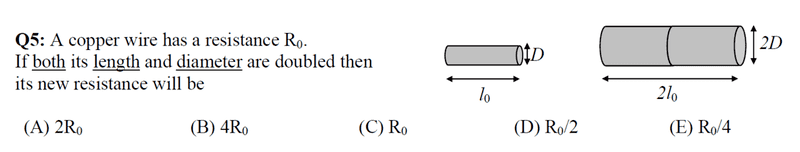# Find the new resistance if its length and D are doubled

Fatima Hasan

## Homework Statementρl = RA
A = π r2

## The Attempt at a Solution

l = RA (ρ is constant)
A1 = π (D/2)2
= (πD2)/4
A2 =π (2D/2)2
= πD2
= A1 /4
l2 = 2*l1
##R=\frac{l}{A}##
R2 = (2l1)/(A1/4)
= 8 l /A
= 8R1

Could someone tell me where is my mistake ?

#### Attachments

Staff Emeritus
Could someone tell me where is my mistake ?

Trying to do this in one bite.

What happens if only its diameter is doubled? (Hint: the area goes up by 4, so it's like having 4 original resistors in parallel)
What happens if only its length is doubled? (Hint: it's like having 2 original resistors in series)
What happens if you do both together?

Fatima Hasan
Trying to do this in one bite.

What happens if only its diameter is doubled? (Hint: the area goes up by 4, so it's like having 4 original resistors in parallel)
What happens if only its length is doubled? (Hint: it's like having 2 original resistors in series)
What happens if you do both together?
(D) . Right ?

Staff Emeritus
Are you asking me or telling me?
(i.e. I'm not going to give you the answer.)

Homework Helper
Look at your calculations again. Is A2 = A1/4?

Fatima Hasan
Look at your calculations again. Is A2 = A1/4?
It should be A2 = A1*4
R = ##\frac{ρ*2l}{4A}##
R = ##\frac{ρl}{2A}##
R2 = R1 /2

•berkeman